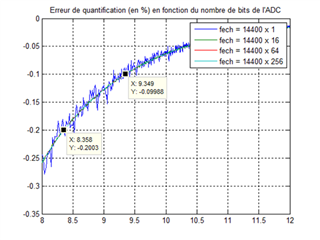If you have a related question, please click the "Ask a related question" button in the top right corner. The newly created question will be automatically linked to this question.

Hello

I have to design an analog to digital convertion stage with a high accuracy (RMS value error < 0.1%) on a large dynamic of 44dB (62.5mA to 10A).

The number of bits necessary to code the full dynamic is log2(10A/62.5mA) = 7.32 bits.

The signal to be mesured being a sine at 50Hz, I have digitalized a normalized 50Hz sinus at a quantification of 2^8 to 2^12 and calculated the RMS value error due to the quantfication :With this, I have determined that the number of bits necessary to respect the accuracy must be > 9.35 bits.

If no considering the noise, the number of bits of the ADC necessary must be 7.32 + 9.35 = 16.67 bits or the SNR must be greater then 16.67 x 6.02 + 1.76 = 102dB.

In your opinion, is this method seems to be correct?

• Hello David,

This is an interesting approach, but I think you are correct.  Another quick check is to assume you want to read a current of 0.001*62.5mArms,  or 62.5uA.  The resulting dynamic range needed would be 20*log(10A/62.5uA)=104dB, but I think your approach is more accurate.

In either case, the ADS8910B is specified for 102.5dB of SNR, and if you need slightly higher SNR, the pin-to-pin ADS8900B can be used with an SNR of 104.5dB.

Regards,
Keith Nicholas